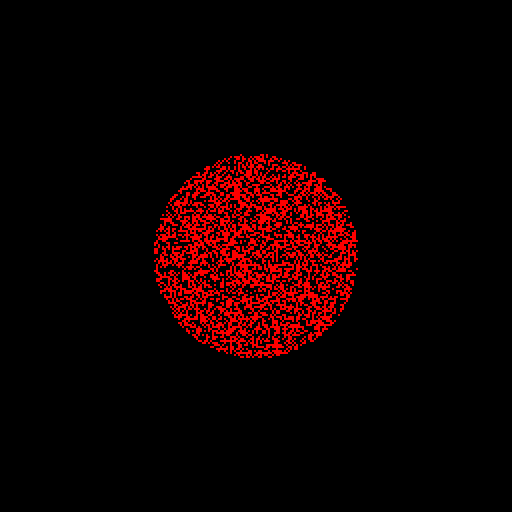# Conway’s game of life

We use a cartesian grid and the generic time loop.

#include "grid/cartesian.h"
#include "run.h"

We need two fields to store the old and new states as well as a field to store the age of each cell.

scalar a[], b[], age[];

The lower-left corner is at (-0.5,-0.5) (the default box size is one) i.e. the domain spans (-0.5,-0.5) (0.5,0.5) and is discretised using 256^2 cells.

int main()
{
origin (-0.5, -0.5);
init_grid (256);

The generic run() function implements the main time loop.

  run();
}

We initialise zeros and ones randomly (the noise() function returns random numbers between -1 and 1) in a circle centered on the origin of radius 2.

event init (i = 0)
{
foreach() {
a[] = (x*x + y*y < sq(0.2))*(noise() > 0.);
age[] = a[];
}
}

## Animation

We generate images of the age field every 5 timesteps for the first 1000 timesteps of the evolution.

event movie (i += 5; i < 1000)
{

We mask out dead cells (i.e. cells for which age is zero).

  scalar m[];
foreach()
m[] = age[] ? 1 : -1;

output_ppm (age, mask = m, n = 512, file = "age.gif", opt = "--delay 1");
}

## Game of life algorithm

event life (i++)
{
foreach() {

We count the number of live neighbors in a 3x3 neighbourhood.

    int neighbors = - a[];
for (int i = -1; i <= 1; i++)
for  (int j = -1; j <= 1; j++)
neighbors += a[i,j];

If a cell is alive and surrounded by 2 or 3 neighbors it carries on living, otherwise it dies. If a cell is dead and surrounded by exactly 3 neighbors it becomes alive.

    b[] = a[] ? (neighbors == 2 || neighbors == 3) : (neighbors == 3);

The age of live cells is incremented.

    age[] = b[]*(age[] + 1);
}

Here we swap the old state (a) with the new state (b).

  swap (scalar, a, b);
}Evolution of the age of cells